# Reasoning Miscellaneous – 2

1) Two Friends H and F are facing each other and standing at a distance of 10 m and faces west and east direction respectively. H starts walking towards north and F starts walking towards south and both are moving 5m. Then H and F take right and left turn respectively and stopped after travelling 5m each. Find the shortest distance between H and F (approximately)?

a) 10 m

b) 12 m

c) 14 m

d) 17 m

e) None of these

c) 14 m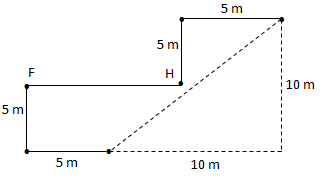=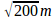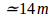D.2-3) Study the following information carefully and answer the questions given below:

Six persons C, D, E, J, K and L are there in a family. There are two fathers, three brothers and a mother in the family. E is the sister of L. D is the brother of K’s husband. J is the father of C and grandfather of L. D is not the brother of J.

Q.9) How is L related to K?

a) Son

b) Daughter

c) Niece

d) Nephew

e) None of these

a) Son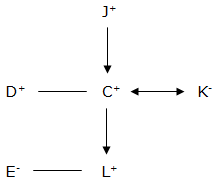3) How many males are there in the family?

a) One

b) Two

c) Three

d) Four

d) Four4-8) Study the following information carefully and answer the questions given below:

I 5 6 @ D # 7 H M E X Q V F 4 & 3 Z Y W 2 8 S 1 N O A *

4) How many such consonants are there in the above arrangement each of which is immediately preceded by a consonant and immediately followed by a vowel?

a) None

b) One

c) Two

d) Three

e) More than three

b) One

Consonant- consonant- vowel

(i) HME

5) Which of the following is fourth to the left of the tenth from the right end of the above arrangement?

a) Q

b) 4

c) 8

d) @

e) Other than given options

b) 4

Fourteenth from the right end = 4

6) How many such numbers are there in the above arrangement each of which is immediately preceded by a consonant?

a) One

b) Two

c) Three

d) Four

e) Other than given options

c) Three

Consonant number

(i) F\$

(ii) W2

(iii) 1S

7) Four of the following five are alike in a certain way based on their positions in the above arrangement and so form a group. Which is the one that does not belong to that group?

a) & Y 2

b) I # @

c) S O *

d) W S N

e) 4 Z W

b) I # @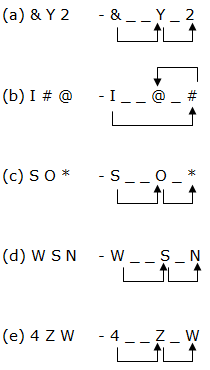8) If all the symbols and numbers from the above arrangement are dropped, which of the following will be tenth from the left end of the above arrangement?

a) Y

b) X

c) Z

d) M

e) Other than given options